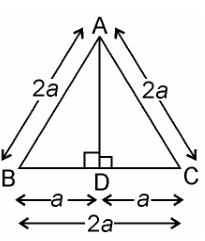# ABC is an equilateral triangle of side 2a. Find each of its altitudes.

Let ABC is an equilateral triangle of each 2a units3a= (AB)2

√3 a= AD ∴ Each of its altitude= √3 a unit